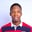a concise shot of dev knowledge
Become a Contributor
Concise shots of dev knowledge

RELATED TAGS

c#
communitycreator

# How to programmatically calculate the area of a cone in C#Theodore Kelechukwu Onyejiaku

## Overview

The surface area of a cone is the summation of the curved area of the cone and the area of the base.

$A=\pi r(r+ \sqrt{h^2+r^2} )$

Where,

• r is the radius of the cone.
• h is the height of the cone.
• π is the mathematical pi.

In C#, we can easily calculate the area of a cone. All we need to do is apply the formula or logic below.

### Syntax

Math.PI * r * (r + Math.Sqrt(h*h + r*r));

### Variables

• Math.PI: This is the pi we know in mathematics.
• r: This represents the radius of the cone.
• h: This represents the height of the cone.

### Return value

The value returned is a double value which represents the surface area of a cone.

### Example

using System;
class HelloWorld
{
// create function to get the areas
static void GetArea(double h, double r){
double area = Math.PI * r * (r + Math.Sqrt(h*h + r*r));
Console.WriteLine("The area of the cone with height "+h+" and radius "+r+" is "+area);
}

// main method
static void Main()
{
// create some radii of some cones
double r1 = 10;
double r2 = 1.5;
double r3 = 4.5;

// create also the heights of each cone
double h1 = 2.2;
double h2 = 5;
double h3 = 20.5;

// get the area of the cones
GetArea(h1, r1);
GetArea(h2, r2);
GetArea(h3, r3);
}
}
Calculating the surface area of a cone in C#

### Explanation

• Line 5: We create a method called GetArea() that takes two parameters, height and radius. It calculates the area of the cone and tells the user the result in the console.
• Lines 14–16: Within the main function, we define the radii of the cones we want to calculate the areas of.
• Lines 19–21: We define the heights of the cones as well.
• Lines 24–26: We invoke the GetArea() method and passed it to the radii and heights we have created. We print the results to the console.

RELATED TAGS

c#
communitycreator

CREATORTheodore Kelechukwu Onyejiaku
RELATED COURSES

View all Courses

Keep Exploring

Learn in-demand tech skills in half the time